mrpolsky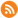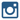# Math Resources Grade 5 Alberta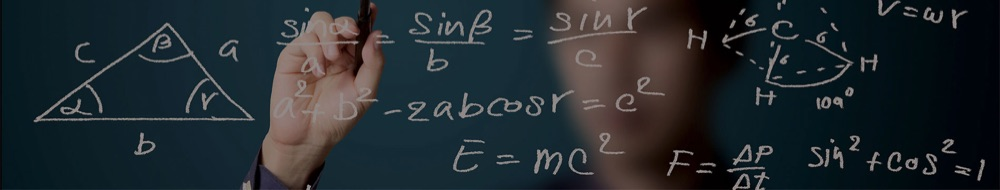MATH

Are you working on your math but can’t remember what that ‘annoying’ math word means? Use this Math Dictionary, organized alphabetically, to help you figure it out. Crisis averted!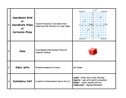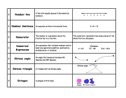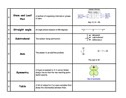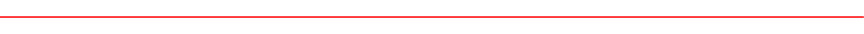Numbers are everywhere in our world. From sports statistics to weather reports, making budgets to getting your allowance. If I want to buy my new Xbox or Playstation, it’s kind of important to be able to a) read the price and b) be able to count my money. In this unit, we will be working with reading and writing numbers, as well as manipulating them in problem solving situations. Operation skills, estimation and everything in between - it’s a lot but hey, that Xbox is awesome and need to count my piggy bank coins!

Reading and writing numbers is huge. If we can’t read the numbers in-front of us then there is no way we can solve the problem. We use the rules of Place Value to get the job done.

We can prove the value of any number and their digits by writing it in any of 1 of 3 ways.

Place Value Games & Interactives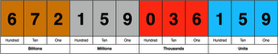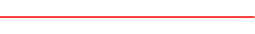When working to solve problems or to help ourselves check our work, we can use Mental Math strategies, aka estimation, to help make things easier.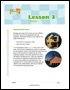Open for Larger

<--    -->

There are many ways to estimate but there are three methods that can be used in any situation.

Estimation Games & Interactives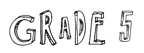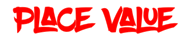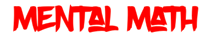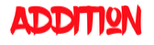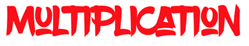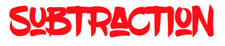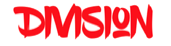Use these links to practise and review how to solve problems with addition.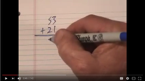Use these links to review how to solve problems with multiplication.

MathsIsFun - Multiplication

Multiplication Games & Interactives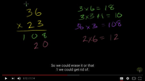Use these links to review how to solve problems with subtraction.

MathsIsFun - Subtraction

Subtraction Games & Interactives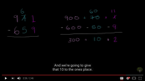Use these links to review how to solve problems with multiplication.

MathsIsFun - Long Division

Long Division Notes & Explanation

Division Games & Interactives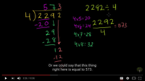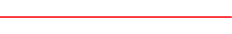Fractions and decimals are everywhere in our lives. You use them all the time and often don’t even realize it. Cooking, money, sports, time, games, and medicine are just a few examples where you use fractions or decimals. As part of this unit, we will be working with these ideas to improve our number sense skills. When did you use fractions last?

MathsIsFun.com [Explanations, videos and resources for all concepts]

Explanations:   Fractions  |  Decimals        Extra Practise:   Fractions  |  Decimals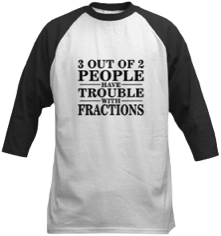The month of March means one thing, and one thing only - March Madness. On top of the slam dunks, the alley-oops and the crossovers, basketball is filled with Math. The best basketball tournament in the world is also the best way to see how fractions, decimals and percents all add up to a NCAA National Champion.What are the odds the Flames will make the playoffs? How likely is it for the Blue Jays to beat the Yankees? The Red Sox? If the students in the class were asked what their favourite sport was, how many would say hockey? These are all questions that could be used in our investigation into Statistics & Probability.

MathsIsFun.com  Probability  |  Probability Line  |  Bar Graphs  |  Line Graphs  |  Pictographs  |  Pie Chart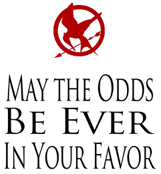Our world is filled with shapes large and small, with angles, lines and different geometric shapes. It’s the oldest math, the most basic math - “What shapes fit together to let me build something?” In this unit, students will be exploring the world of angles, polygons, volume and area, as well as how we can measure these. Lastly, students will be exploring 2D and 3D shapes with their different characteristics.

Geometry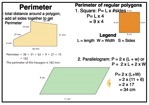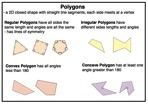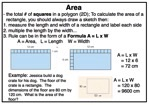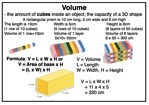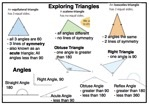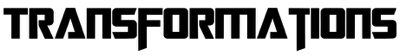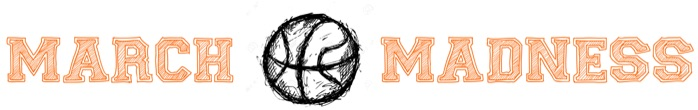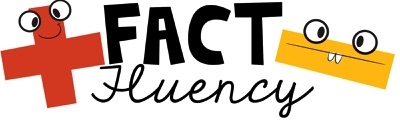Check out these websites or games to practise your math facts.

Tux of Math Command (Must Download)  |  Math Flash Cards  |  Math U See Drills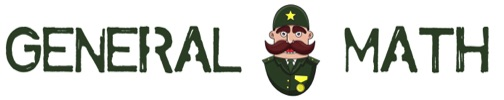Math is everywhere and so are the places you can go to practise and learn. In grade five we have lots of different resources that we can use. These are some links that can help you with all areas of math. You can thank the General for all these helpful sites. Just make sure you do what he says!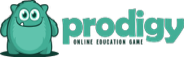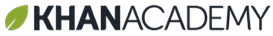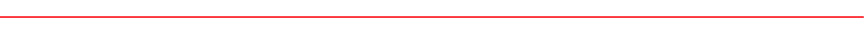Khan Academy: Pixar in a Box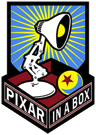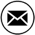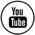Probability InteractivesProbability

StatisticsMeasurement

Geometry